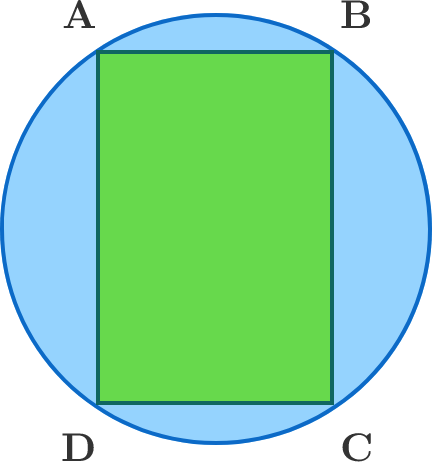Rectangle Area?

Geometry Level 1

Rectangle ABCD is inscribed in a circle O picture below. If the circumference of circle is 5*PI and AD=4 than what is the area of the rectangle ABCD?×